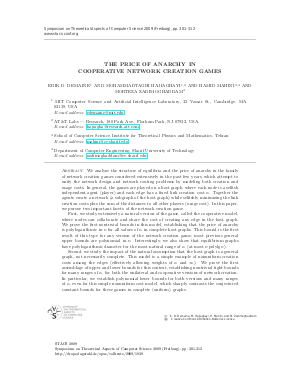Document# The Price of Anarchy in Cooperative Network Creation Games## File

LIPIcs.STACS.2009.1839.pdf
• Filesize: 215 kB
• 12 pages

## Cite As

Erik D. Demaine, MohammadTaghi Hajiaghayi, Hamid Mahini, and Morteza Zadimoghaddam. The Price of Anarchy in Cooperative Network Creation Games. In 26th International Symposium on Theoretical Aspects of Computer Science. Leibniz International Proceedings in Informatics (LIPIcs), Volume 3, pp. 301-312, Schloss Dagstuhl - Leibniz-Zentrum für Informatik (2009)
https://doi.org/10.4230/LIPIcs.STACS.2009.1839

## Abstract

We analyze the structure of equilibria and the price of anarchy in the family of network creation games considered extensively in the past few years, which attempt to unify the network design and network routing problems by modeling both creation and usage costs. In general, the games are played on a host graph, where each node is a selfish independent agent (player) and each edge has a fixed link creation cost~$\alpha$. Together the agents create a network (a subgraph of the host graph) while selfishly minimizing the link creation costs plus the sum of the distances to all other players (usage cost). In this paper, we pursue two important facets of the network creation~game. First, we study extensively a natural version of the game, called the cooperative model, where nodes can collaborate and share the cost of creating any edge in the host graph. We prove the first nontrivial bounds in this model, establishing that the price of anarchy is polylogarithmic in $n$ for all values of~$\alpha$ in complete host graphs. This bound is the first result of this type for any version of the network creation game; most previous general upper bounds are polynomial in~$n$. Interestingly, we also show that equilibrium graphs have polylogarithmic diameter for the most natural range of~$\alpha$ (at most $n \mathop{\rm polylg}\nolimits n$). Second, we study the impact of the natural assumption that the host graph is a general graph, not necessarily complete. This model is a simple example of nonuniform creation costs among the edges (effectively allowing weights of $\alpha$ and~$\infty$). We prove the first assemblage of upper and lower bounds for this context, establishing nontrivial tight bounds for many ranges of~$\alpha$, for both the unilateral and cooperative versions of network creation. In particular, we establish polynomial lower bounds for both versions and many ranges of~$\alpha$, even for this simple nonuniform cost model, which sharply contrasts the conjectured constant bounds for these games in complete (uniform) graphs.

## Metrics

• Access Statistics
• Total Accesses (updated on a weekly basis)
0